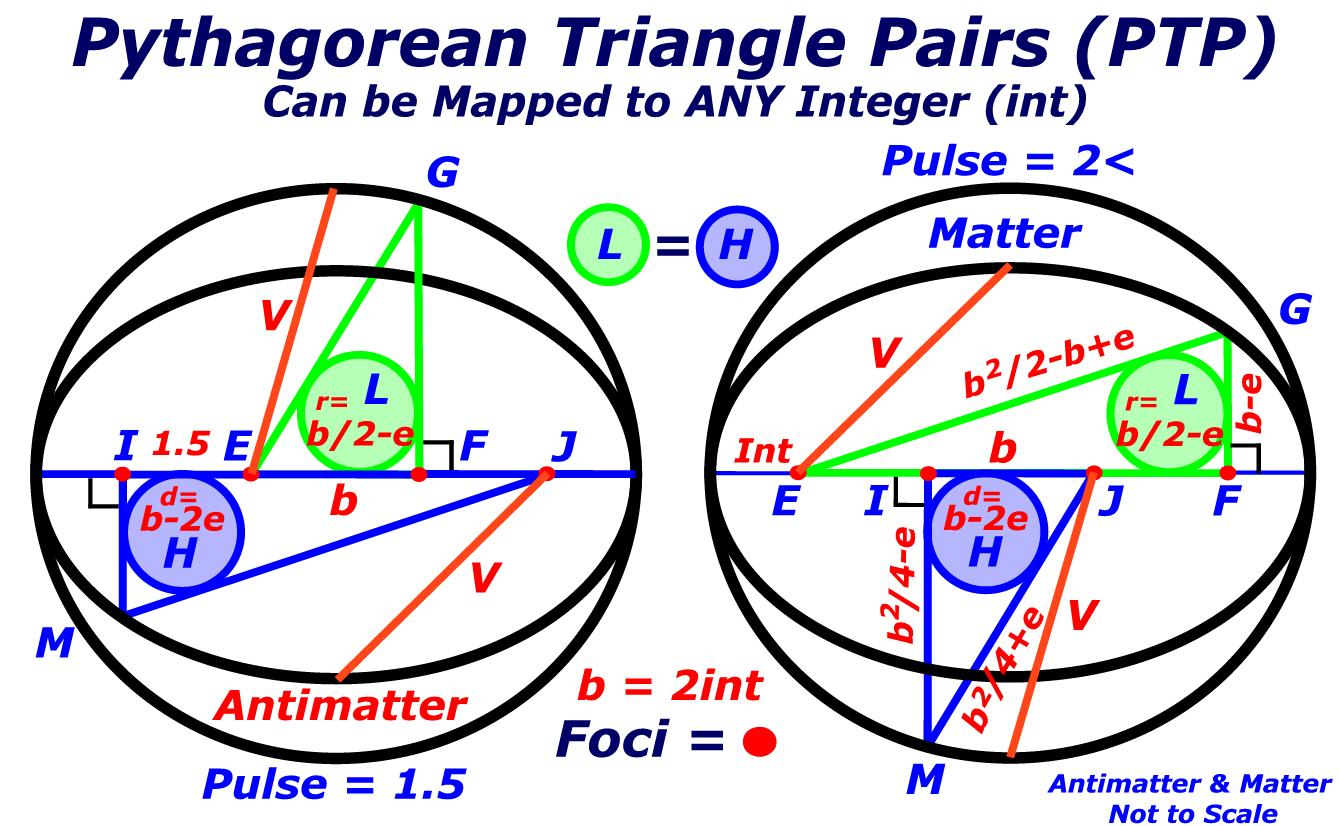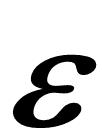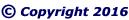"CLICK" below images; or, above blue ellipse to navigate.
 Emergent Ellipsoid GeometryDF = Vector = x2
 AB = Major Diameter = 2x2
 DE = Minor Diameter = SqRt(x4–2x3+x2)
 FG = wave = 2(x2–x)
 FH = hypotenuse = 2x2–2x+GH = radius = 2x–HK = Vector radius = x2–Extend the Minor Diameter, Line DE, as required. Lines CI and CJ equal Lines AF and BG. Draw Lines IK and JK such that they equal the Vector.
 "CLICK" above images; or, below blue ellipse to navigate.One must continuously ask, Why? Why? Why?
 Proof of One Epsilon equals One Natural Function revised Fibonacci Sequence Elliptical Constant Duality of Infinity